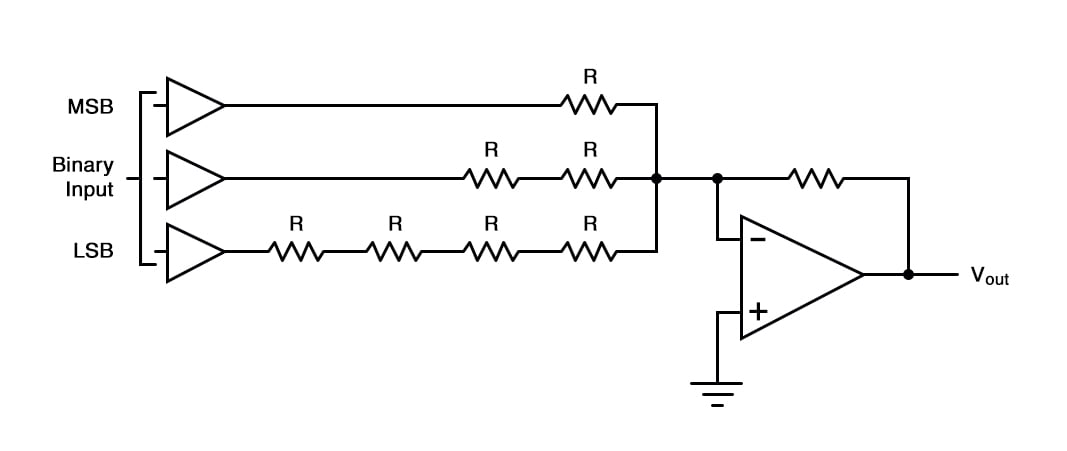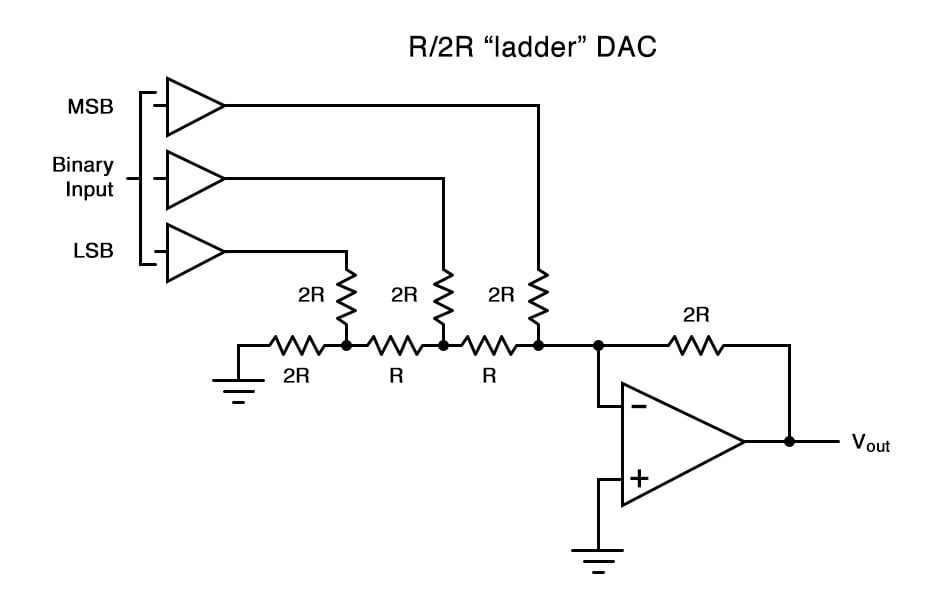# The R/2R DAC (Digital-to-Analog Converter)

## Chapter 13 - Digital-Analog Conversion

The R/2R DAC circuit is an alternative to the binary-weighted-input (R/2nR) DAC which uses fewer unique resistor values.

### R/2R DAC vs. R/2nR DAC

A disadvantage of the former DAC design was its requirement of several different precise input resistor values: one unique value per binary input bit. Manufacture may be simplified if there are fewer different resistor values to purchase, stock, and sort prior to assembly.

Of course, we could take the binary-weighted-input DAC circuit and modify it to use a single input resistance value, by connecting multiple resistors together in series:Unfortunately, this approach merely substitutes one type of complexity for another: volume of components over diversity of component values. There is, however, a more efficient design methodology.

### What Is an R/2R Ladder DAC?

By constructing a different kind of resistor network on the input of our summing circuit, we can achieve the same kind of binary weighting with only two kinds of resistor values, and with only a modest increase in resistor count.  This “ladder” network looks like this:Mathematically analyzing this ladder network is a bit more complex than for the previous circuit, where each input resistor provided an easily-calculated gain for that bit.

For those who are interested in pursuing the intricacies of this circuit further, you may opt to use Thevenin’s theorem for each binary input (remember to consider the effects of the virtual ground), and/or use a simulation program like SPICE to determine circuit response. Either way, you should obtain the following table of figures:

------------------ | Binary | Output voltage | -------- | 000 | 0.00 V |
------------------ | 001 | -1.25 V | ------------------ | 010 | -2.50 V |
------------------ | 011 | -3.75 V | ------------------ | 100 | -5.00 V |
------------------ | 101 | -6.25 V | ------------------ | 110 | -7.50 V |
------------------ | 111 | -8.75 V | ------------------


 Binary Output Voltage Binary Output Voltage 001 -1.25 V 000 -0.00 V 011 -3.75 V 010 -2.50 V 101 -6.25 V 100 -5.00 V 111 -8.75 V 110 -7.50 V

RELATED WORKSHEET:

• Share
Published under the terms and conditions of the Design Science License